• 无向图 有向图 有向网 无向网 深度优先遍历 Ｃ语言　用户操纵界面
• 何为（Graph） 形似如下抽象结构： 这看起来的确有点抽象￣□￣｜｜。。 我们再看一张： ...这是一张二叉树，我在之前的基础上减去了几根“联系”就变成了树。...所以在一定程度上，可以把理解为...DFS深度优先遍历
何为图（Graph）
形似如下抽象结构：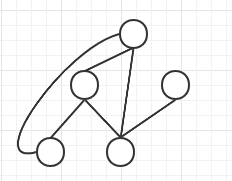这看起来的确有点抽象￣□￣｜｜。。

我们再看一张：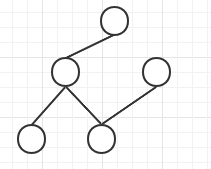这是一张二叉树，我在之前图的基础上减去了几根“联系”就变成了树。

所以在一定程度上，可以把图理解为树的延伸（图进一步的打破了树的“规矩”）。
程序中的“图”
图的存储
需要一个集合（Set）来存储我们的节点元素。需要一个映射（HashMap）来存储节点是否被访问过。需要一个 HashMap<T, ArrayList> 来存储节点间的通路。
如下图所示：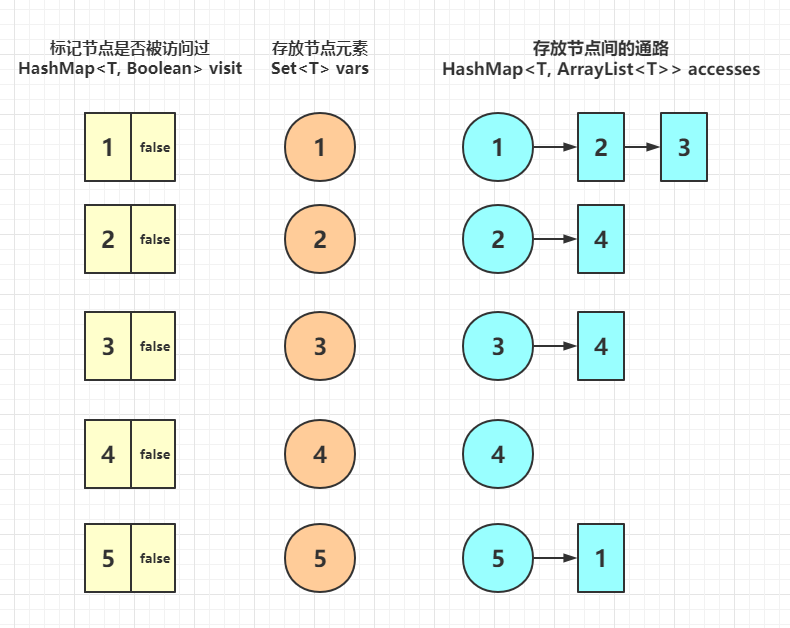代码实现

DFS深度优先遍历算法也在里面。


import java.util.*;

/**
* @ClassName ArrayGraph
* @Description 自定义“有向图”class，不允许有重复的元素
* @Author SkySong
* @Date 2021-05-16 17:14
*/
public class ArrayGraph<T> {
//存放节点元素
private Set<T> vars;
//标记节点是否被访问过
private HashMap<T, Boolean> visit;
//节点间的通路
private HashMap<T, ArrayList<T>> accesses = new HashMap<>();

/**
* 清空访问
*/
public void clearVisit() {
try {
vars.forEach((k) -> visit.put(k, false));
} catch (Exception e) {
e.printStackTrace();
}
}

/**
* 初始化节点集合
*
* @param vars 节点集合
*/
public ArrayGraph(Set<T> vars) {
this.vars = vars;
visit  = new HashMap<>();
clearVisit();
}

/**
* 添加节点间通路
*
* @param from 出发节点
* @param to   目的节点
*/
public void addAccess(T from, T to) {
if (!vars.contains(from) && !vars.contains(to)) {
return;
}
ArrayList<T> ts = accesses.get(from);
if (ts == null) {
ts = new ArrayList<>();
}
accesses.put(from, ts);
}

/**
* DFS 深度优先遍历
*
* @return 图 “变” 数组
*/
return null;
}
//创建一个list，用来存放最终的有序 序列
ArrayList<T> list = new ArrayList<>();
if (ts == null || ts.isEmpty()){
return list;
}
Stack<T> stack = new Stack<>();
while (!stack.isEmpty()){
int index = 0;
for (T t : ts) {
//如果此节点已经访问过了，我们就不做任何操作
if (visit.get(t)){
continue;
}
//如果此节点没有访问过，访问之
//改变节点访问状态
visit.put(t,true);
//探寻此节点的下一层“通路”
ts = accesses.get(t);
if (ts != null){
//如果此节点下一层有“通路”，便将此节点放入栈中
stack.push(t);
//并改变标志位，跳过出栈操作
index++;
}
break;
}
//如果此节点没有下一层可以访问，则触发出栈操作，去上一层寻找
if (index == 0){
T pop = stack.pop();
ts = accesses.get(pop);
}
}
return list;
}
}


针对这个DFS，我们大致阐述一下思路。

首先明确一点，图的遍历是需要确定一个起始节点的。（这也是我们这个DFS方法的参数）。

首先我们需要一个栈，这个栈是完成我们“深度优先”的主要工具

何为“深度优先”，当我们在访问过程中，遇到分支了，优先选择下一层的分支进行访问。

我们利用栈“先进后出”的特性，将当前节点的“上级”们先逐层保存起来。确保我们能向上逐层找到他们。
我们开始进行访问，每层访问一个节点便向下层访问，当我们发现不能向下了（与当前节点相连的节点都被访问过了），就往上倒一层，然后重复上述过程。
测试
public static void main(String[] args) {
ArrayGraph<Integer> graph = new ArrayGraph<>(Sets.newHashSet(1,2,3,4,5));

System.out.println(graph.DFSOrder(5).toString());
}

结果：

[5, 1, 2, 4, 3]

附上图，大家自行脑补验证：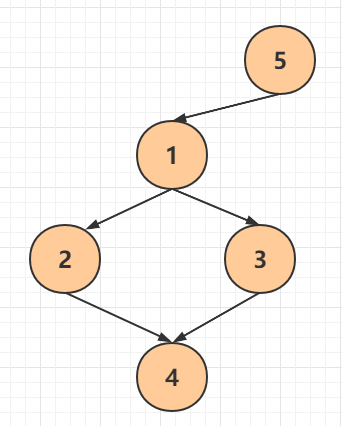拓展
本例说的是有向图，其实也可以当做无向图来用：

当我们在添加“通路”时，顺便把反向的也添加进去，便可以实现了。

本例说的是不允许重复元素，其实可以变向思考一下这个问题。

在图的实际应用中，元素经常是引用对象，是比较复杂的数据结构，也许他们里面的内容相同，但他们的引用地址不同，所以在一定程度上“相同”也是可以实现的。

That’s all,thank you ! ! !
展开全文java 数据结构
• 创建（无）向图深度优先遍历，广度优先遍历 代码实现： #include<stdio.h> #include<stdlib.h> #include<string.h> #define MaxVertexNum 100 #define MaxSize 10 //顶点数目的最大值 ...
邻接表存储结构:
创建有（无）向图，深度优先遍历，广度优先遍历
代码实现：
#include<stdio.h>
#include<stdlib.h>
#include<string.h>
#define MaxVertexNum 100
#define MaxSize 10
//顶点数目的最大值
bool visitedBFS[MaxVertexNum];//用于广度优先遍历的访问标记数组
bool visited[MaxVertexNum];//用于深度优先遍历的访问标记数组
typedef  char VertexType;//顶点数据类型
typedef int EdgeType;//带权图中边上权值的数据类型 //这样写有便于数据类型的修改，就是在这修改数据类型就可以，低耦合
//邻接表
//边表结点
typedef struct ArcNode{
ArcNode *next;
}ArcNode;
//顶点表结点
typedef struct VNode{
VertexType data;
ArcNode *first;
//图
typedef struct ALGraph{
int vexnum,arcnum;//顶点数弧数
}ALGraph;

//创建有（无）向图
void createGraph(ALGraph &g){
printf("请输入顶点数和边数：");
int n,m;
scanf("%d%d",&n,&m);
g.vexnum=n;
g.arcnum=m;
for(int i=0;i<g.vexnum;i++){//初始化顶点
g.vertices[i].data=i;
g.vertices[i].first=NULL;
}
printf("请输入边的信息(顶点是0~n-1)\n");
int x,y;
for(int i=0;i<g.arcnum;i++){
scanf("%d%d",&x,&y);
ArcNode *p =(ArcNode*)malloc(sizeof(ArcNode));
p->next=g.vertices[x].first;//将该边表结点的next域指向顶点表结点的first域
g.vertices[x].first=p;//将顶点表的first域指向p
//如果是无向图的话应该把<y,x>也存储起来
ArcNode *q=(ArcNode*)malloc(sizeof(ArcNode));
q->next=g.vertices[y].first;
g.vertices[y].first=q;

}
}

//深度优先遍历图,从第v个顶点开始遍历
void DFS_Graph(ALGraph g,int v){
visited[v]=true;
printf("%d  ",g.vertices[v].data);
ArcNode *p=g.vertices[v].first;
int w;
while(p!=NULL){
if(!visited[w]){
DFS_Graph(g,w);//递归深度遍历
}
p=p->next;
}
}

//广度优先遍历
//借助队列
//从v开始遍历
void BFS_Graph(ALGraph g,int v){
visitedBFS[v]=true;
ArcNode *p;
printf("%d  ",g.vertices[v].data);
int que[MaxSize];
int front=0,rear=0;
rear=(rear+1)%MaxSize;
que[rear]= v;
int j;
while(rear!=front){//当队列不空的时候

front=(front+1)%MaxSize;//出队
j =que[front];
p =g.vertices[j].first;
while(p!=NULL){

rear=(rear+1)%MaxSize;
}
p=p->next;//用的是邻接表，用p-next就可访问同层的相邻结点

}

}

}

int main(){
ALGraph g;
createGraph(g);
printf("深度优先遍历顺序：\n");
DFS_Graph(g,2);
printf("\n广度优先遍历顺序：\n");
BFS_Graph(g,0);

}


代码运行截图：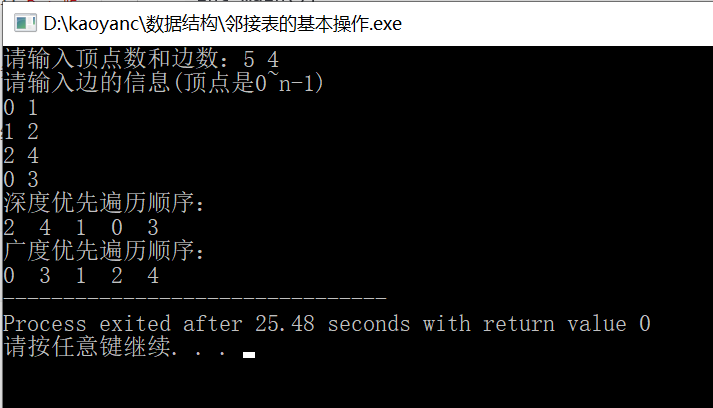展开全文邻接表
• //用邻接矩阵存储有向图深度优先遍历 #define MaxSize 10 #define inf 0x3f3f3f bool book[MaxSize]; int n,m; //顶点数 边数 struct MGraph{ //定义图 int edge[MaxSize][MaxSize]; //存储边 int vexnum,...
实现代码：
#include<iostream>
#include<queue>
using namespace std;
//用邻接矩阵存储有向图的深度优先遍历

#define MaxSize 10
#define inf 0x3f3f3f

bool book[MaxSize];
int n,m; //顶点数 边数

struct MGraph{ //定义图
int edge[MaxSize][MaxSize]; //存储边
int vexnum,arcnum; //定点数 边数
};

int FirstNeighbor(MGraph G,int x){

for(int i=0;i<n;i++){
if(G.edge[x][i]=1)
return i;
}

return -1;
}

int NextNeighbor(MGraph G,int x,int j){

for(int i=j+1;i<n;i++){
if(G.edge[x][i]==1)
return i;
}

return -1;
}

//深度优先遍历
void dfs(MGraph G,int x){

cout<<x<<" "; //访问队头元素
book[x]=true;

for(int i=FirstNeighbor(G,x);i>=0;i=NextNeighbor(G,x,i)){
if(book[i]==false){
book[i]=true;
dfs(G,i);
}
}

}

int main(){

cin>>n>>m; //输入顶点数和边数

MGraph G; //定义一个图

//初始化图
G.vexnum=n; //顶点数
G.arcnum=m; //边数
for(int i=0;i<n;i++){
for(int j=0;j<n;j++){
if(i==j){
G.edge[i][j]=0;
}
else{
G.edge[i][j]=inf;
}

}
}

//创建有向图
for(int i=0;i<m;i++){
int x,y; //起点 终点
cin>>x>>y;
G.edge[x][y]=1; //初始化边
}

//初始化标记数组
for(int i=0;i<n;i++)
book[i]=false;

//当图不连通时，用for循环能遍历图的所有连通分量，求出图的所有连通分量的广度优先遍历
for(int i=0;i<n;i++){
if(book[i]==false)
dfs(G,0); //深度优先遍历
}

return 0;
}

/*
输入样例：
5 5
1 0
0 2
4 3
3 1
2 4

输出样例
0 2 4 3 1
*/

运行结果：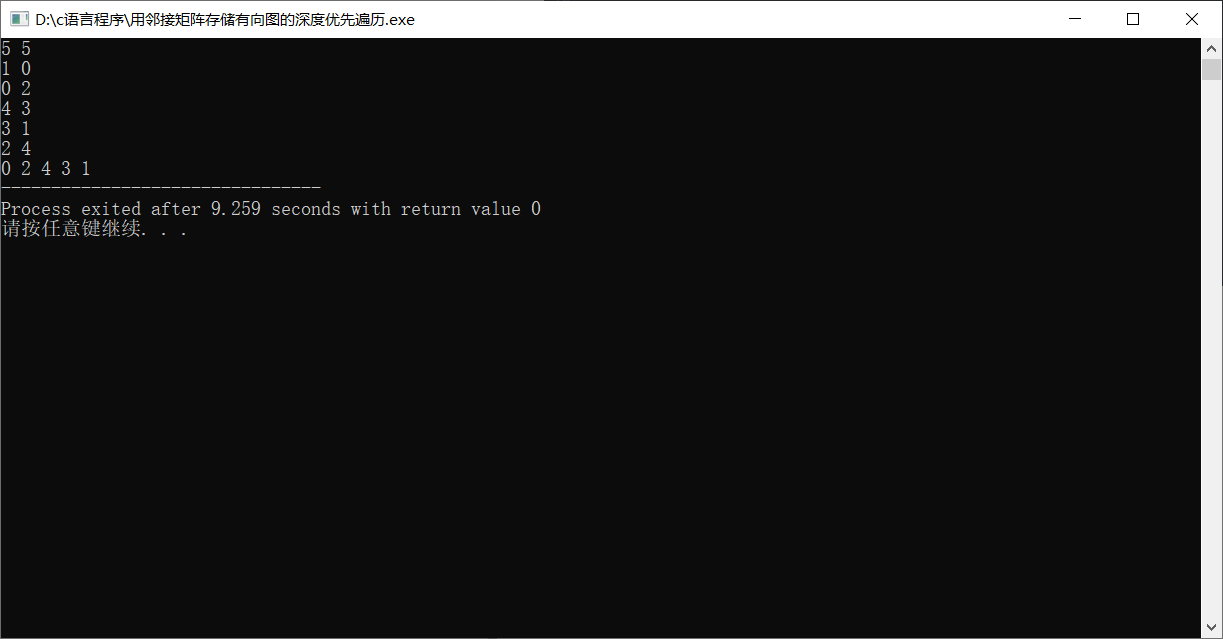展开全文数据结构 算法
• ## 图深度优先遍历

千次阅读 2020-12-03 16:15:46
编写程序对给定的有向图（不一定连通）进行深度优先遍历，图中包含n个顶点，编号为0至n-1。本题限定在深度优先遍历过程中，如果同时出现多个待访问的顶点，则优先选择编号最小的一个进行访问，以顶点0为遍历起点。 ...
编写程序对给定的有向图（不一定连通）进行深度优先遍历，图中包含n个顶点，编号为0至n-1。本题限定在深度优先遍历过程中，如果同时出现多个待访问的顶点，则优先选择编号最小的一个进行访问，以顶点0为遍历起点。
输入格式:
输入第一行为两个整数n和e，分别表示图的顶点数和边数，其中n不超过20000，e不超过50。接下来e行表示每条边的信息，每行为两个整数a、b，表示该边的端点编号，但各边并非按端点编号顺序排列。
输出格式:
输出为一行整数，每个整数后一个空格，即该有向图的深度优先遍历结点序列。
输入样例1:
3 3
0 1
1 2
0 2
输出样例1:
0 1 2 
输入样例2:
4 4
0 2
0 1
1 2
3 0
输出样例2:
0 1 2 3 

#include<bits/stdc++.h>
using namespace std;
typedef long long int ll;
const int N=20005;
vector<int> a[N];
int main(){
int n,e,x,y;
cin>>n>>e;
for(int i=0;i<e;i++){
cin>>x>>y;
a[x].push_back(y);
}
for(int i=0;i<n;i++){
sort(a[i].rbegin(),a[i].rend());
}
vector<int> v(n,0);
stack<int> st;
for(int i=0;i<n;i++){
st.push(i);
while(!st.empty()){
int w=st.top();
st.pop();
if(!v[w]){
cout<<w<<" ";
v[w]=1;
int sz=a[w].size();
for(int j=0;j<sz;j++){
if(!v[a[w][j]]) st.push(a[w][j]);
}
}
}
}
return 0;
}


展开全文• = 9999999 && book[j] == 0) //判断当前顶点cur到顶点j是否路，并判断顶点j是否在已走过的路径中 { book[j] = 1; //标记城市j已经在路径中 dfs(j,dis+e[cur][j]); //从顶点j在出发，继续寻找目标顶点 ...
• 一、深度优先遍历DFS （一）深度优先遍历 bool visited[MAX_VERTEX_NUM]; //访问标记数组,????初始值为false void DFS(Graph G, int v){ //从顶点v出发,深度优先遍历图G visit(v); //访问顶点v visited[v]...DFS算法
• C++实现，数据结构，的邻接矩阵表示，深度优先遍历，广度优先遍历，DFS，BFS，为什么要五十个字才能上传啊
• 有向图的实现（Directed Graph） 有向图的实现有两种，一种是用矩阵（Matrix）的形式来实现，另一种是用链表（List）的形式来实现。 如果我们使用矩阵来实现有向图，来看一个例子： 每行代表相应的顶点，如果M[i][j...java 数据结构 算法
• //用邻接表存储有向图深度优先遍历 #define MaxSize 10 #define inf 0x3f3f3f int n,m; //顶点数 边数 bool book[MaxSize]; //标记数组 queue<int>q; //边 struct ArcNode{ int data; ArcNode *next; }...数据结构 算法
• 遍历深度优先遍历无向图的深度优先遍历图解有向图深度优先遍历图解广度优先遍历无向图的广度优先遍历图解有向图的广度优先遍历图解 图的遍历，所谓遍历，即是对结点的访问。一个图有那么多个结点，如何遍历这些...
• 本文介绍使用java.util.*包中的HashMap 和 LinkedList 以及 ArrayList类快速实现一个有向图，并实现有向图深度优先遍历算法。 如何构造图？ 本文根据字符串数组来构造一个图。图的顶点标识用字符串来表示，如果...
• 全部功能采用Matlab编写，程序的功能是寻找从出发点到目的地的全部可行路径，最后只显示了最佳和最劣路径的动画效果，对每一步的移动进行了动画演示。
• 主要介绍了C++实现的邻接矩阵存储和广度、深度优先遍历,实例分析了C++实现的遍历技巧,非常具有实用价值,需要的朋友可以参考下
• 1. 输入一个有向图的顶点数 n 和边数 e，设图中顶点编号为 1 到 n， ...2. 实现图的深度优先遍历和广度优先遍历，输入顶点序号 v，给出 1 中有向图 自 v 开始的深度优先遍历序列和广度优先遍历序列c++ c语言
• #File Name : 深度优先遍历.py #1 利用栈实现 #2 从源节点开始把节点按照深度放入栈，然后弹出 #3 每弹出一个点，把该节点下一个没有进过栈的邻接点放入栈 #4 直到栈变空 def dfs(node): if node is None: ...
• 深度优先遍历(Depth-First Traversal) ...若w未曾访问过，则以w为新的出发点继续进行深度优先遍历，直至中所有和源点v路径相通的顶点(亦称为从源点可达的顶点)均已被访问为止。若此时中仍
• 通过键盘输入图的顶点，以及每一条边的两个顶点，从而建立无向图。实现无向图深度优先遍历算法。要求以用户给定的结点为起始点，显示深度优先遍历次序。
• 有向图： 在有向图中，结点对,y＞是有序的，结点对,y＞称为从结点x到结点y的一条有向边，因此，,y＞与,x＞是两条不同的边。有向图中的结点对,y＞用一对尖括号括起来，x是有向边的始点，y是有向边的终点，有向图中的...广度优先遍历
• 遍历的一种基本操作，的许多其它操作都是建立在遍历操作的基础之上。 由于结构本身的复杂性，所以遍历操作也较复杂，主要表现在以下四个方面： ① 在结构中，没有一个“自然”的首结点，中任意...广度优先遍历
• 深度优先遍历（C语言数据结构）C语言的代码，可用visual C++进行编译
• 使用字典存储下面这个结构，编写的遍历算法，对给定进行深度优先遍历。 Hint: 结点作为字典的Key，结点的邻居作为字典的Value，从而构成一个。 递归实现深度优先遍历(DFS) 1、字典graph存储,存储顺序...python 数据结构 算法 dfs
• 请问根据图中邻接表画的有向图遍历结果最后需不需要带c呢？如果带的话因为什么，如果不带的话又因为什么？求解答！c语言 数据结构
• 对于下图所示的有向图(访问顺序按序号从小到大)，试写出： (1) 从顶点①出发进行深度优先搜索所得到的深度优先生成树；(2) 从顶点②出发进行广度优先搜索所得到的广度优先生成树。 package com.test.tree; ...
• c++ 数据结构 有向图深度 广度 遍历 全代码 参考资料 任燕版 数据结构
• 深度优先遍历 （1）从中某个初始顶点v出发，首先访问初始顶点v。 （2）选择一个与顶点v相邻且没被访问过的顶点w，再从w出发进行深度优先搜索，直到中与当前顶点v邻接的所有顶点都被访问过为止。　 （3） 利用...数据结构 算法 链表
• c语言实现的邻接表连通图深度优先遍历，包括图的邻接表建立，图的深度优先遍历
• - PAGE PAGE 2 欢迎下载 实验报告 学院系名称计算机与通信工程学院 姓名 学号 专业 计算机科学与技术 班级 实验项目 实验四深度优先与广度优先遍历 课程名称 数据结构与算法 课程代码 0661913 实验时间 2019年4...
• 1、深度优先遍历 DFS:Depth First Search 算法思想：1、以一个未被访问过的顶点作为起始顶点，沿当前顶点的的边走向未被访问过的顶点； 2、执行1步骤后，当该分支结点都被访问后，则返回到上一个顶点，继续...c语言 c++...